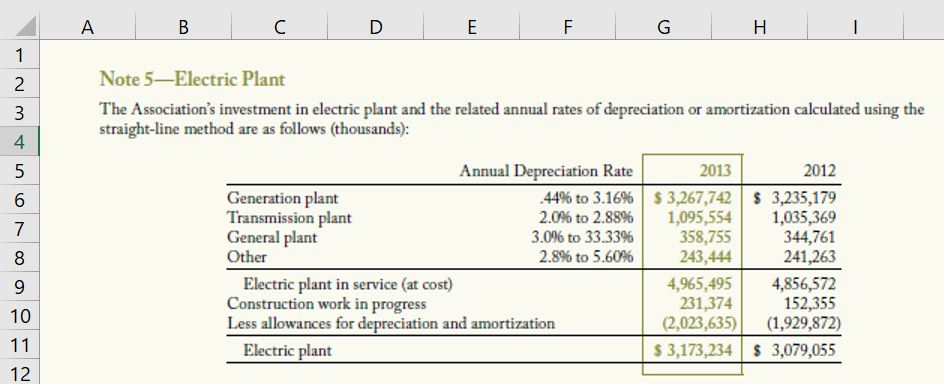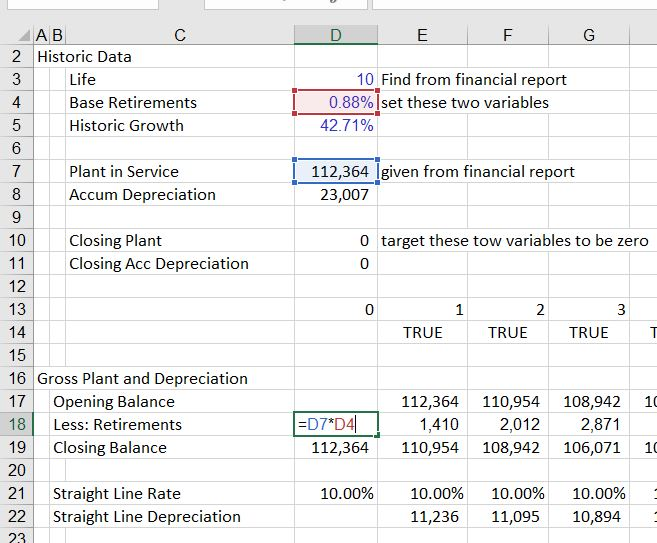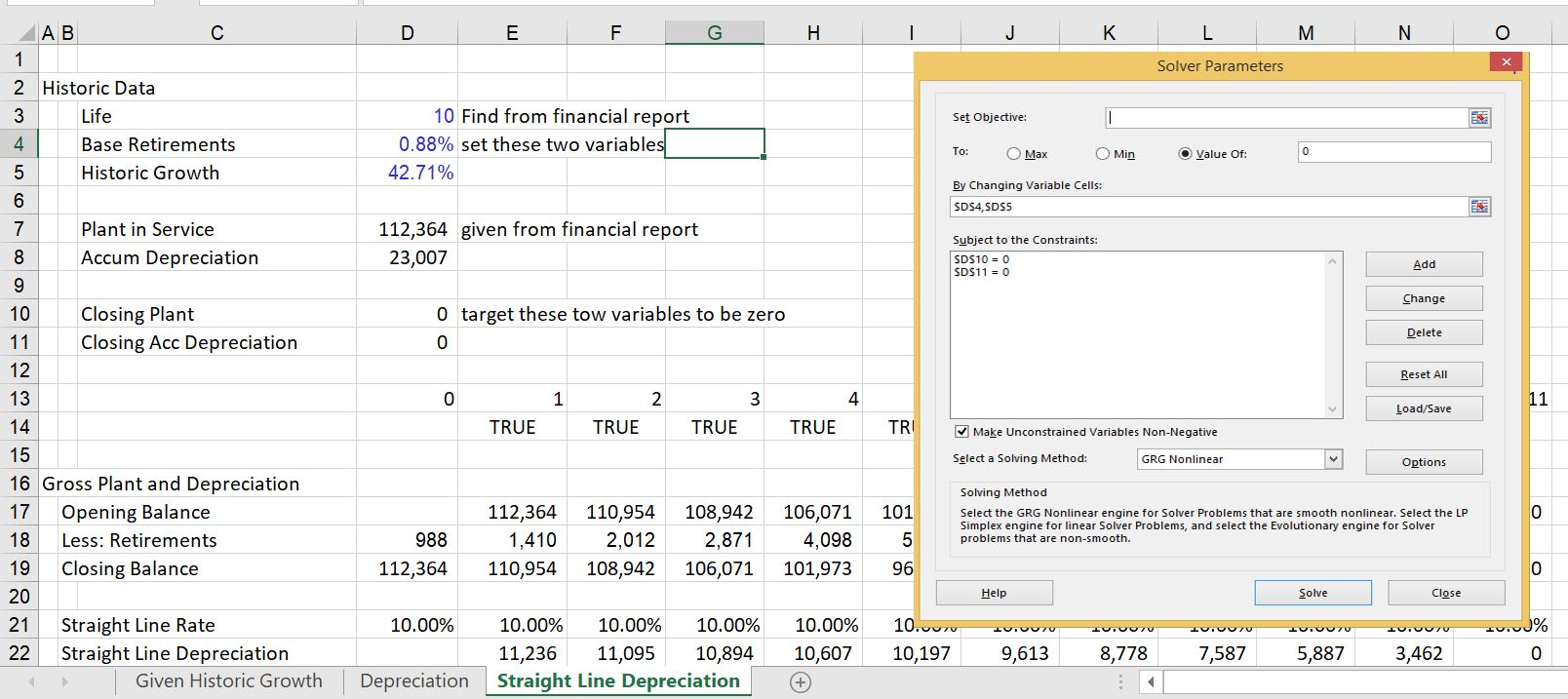# Computing the Implied Retirements from Financial Statement Information

This file below demonstrates how to simulate retirements on existing assets so that you can model the depreciation on existing assets separately from new assets. The growth rate in retirements can be established from the accumulated depreciation and the base can be established from the amount of retirements necessary to make the balance of accumulated depreciation equal the gross plant by the end of plant life. To do this I set up a separate little page and then use the SOLVER function.

An effective way to deal with depreciation, is to first separate depreciation into depreciation related to new assets and existing assets. Depreciation on new assets can be computed using gross plant and reflecting retirements on the new assets. If the depreciation rate is not straight line, you can use a vintage depreciation function. The problem with existing depreciation is computing implied retirements. This can be accomplished by using the solver tool as shown in the videos and files below. The videos and files demonstrate how to make functions and the problems that can arise from incorrectly modelling depreciation.

You can separate existing depreciation from new depreciation. Then, you can evaluate the retirements on existing depreciation using the process below.

The best way I have found to do this is to first find the historic growth rate in capital expenditures as well as a starting point for the retirements.

Use the Solver to Find the Base Level of Retirements and the Historic Growth Rate

To do this:

Step 1: Derive the plant life for one category of assets or the assets in aggregate.

Step 2: Set-up a schedule with gross plant that has balance from the balance sheet. Include retirements as part of the schedule.

Step 3: Set-up a schedule with the accumulated depreciation that also includes retirements.

Step 4: Put an equation with a base level of retirements and a growth rate to compute the amount of period by period retirements.

Step 5: Use the Solver feature to derive the base level of retirements and the growth rate by setting the closing balance to zero.

The way this works is shown in the screenshots below.  The first screenshot demonstrates how you can set-up the balance of plant and the balance of accumulated depreciation.  This balance is only for the existing plant.The second screenshot demonstrates where you can find information on the balance of plant and the balance of depreciation.  With the balance of gross plant, you can divide the depreciation expense by the gross plant balance and derive the average depreciation rate as well as the plant life.The next two screenshots illustrate how to set-up an equation for retirements.  If the historic growth in capital expenditures has been constant, then the retirements should also grow at a constant rate associated with the existing plant.  Of course this is a simplistic assumption and you could make some kind of other equation.  The screenshot below illustrates how the base level of retirements as a percent of the gross plant provides the starting point for retirements.  This is an arbitrary number that will result in the total amount of plant being retired.The screenshot below illustrates a few things. First, the screenshot illustrates how you can create the equation for retirements that grow over time.  Second, the screenshot demonstrates that at the end of the life of the existing assets, the gross plant and the accumulated depreciation go down to zero.  The task is to find the growth rate and the base level that results in the zero balances.  The screenshot also illustrates how you can create a TRUE/FALSE switch that allows you to change the life of the plant. Note that you can make multiple classes of assets and compute the retirement amount for different classes.The final screenshot illustrates how to use the Solver tool to compute the base retirement percentage and the growth rate.  In the solver, the changing cells is both of the two inputs.  The constraint is that the final balance of both the accumulated depreciation and the closing balance is zero.  The closing balance is shown as a separate calculation in D10 and D11. The equations in these cells could use the Sumif function.The file that contains an illustration of how to use the solver so you can find depreciation and retirements is available for downloading below.